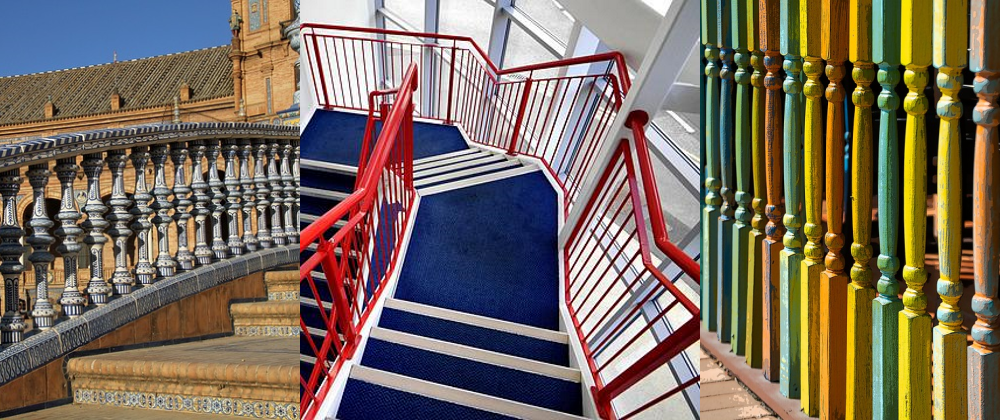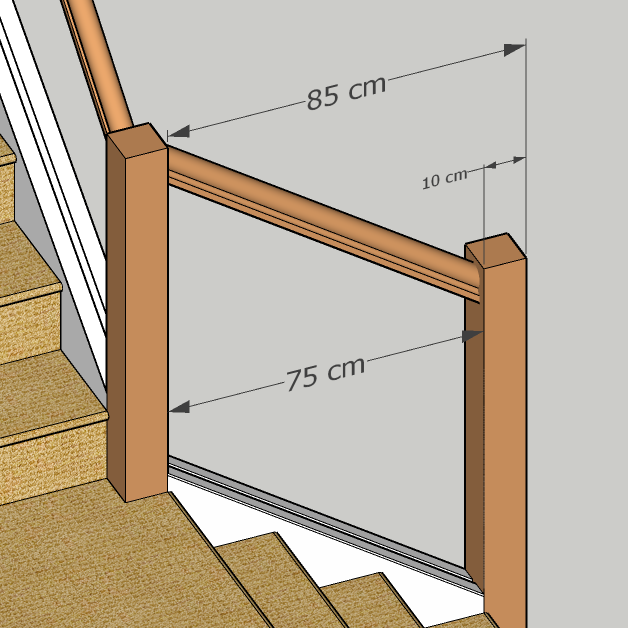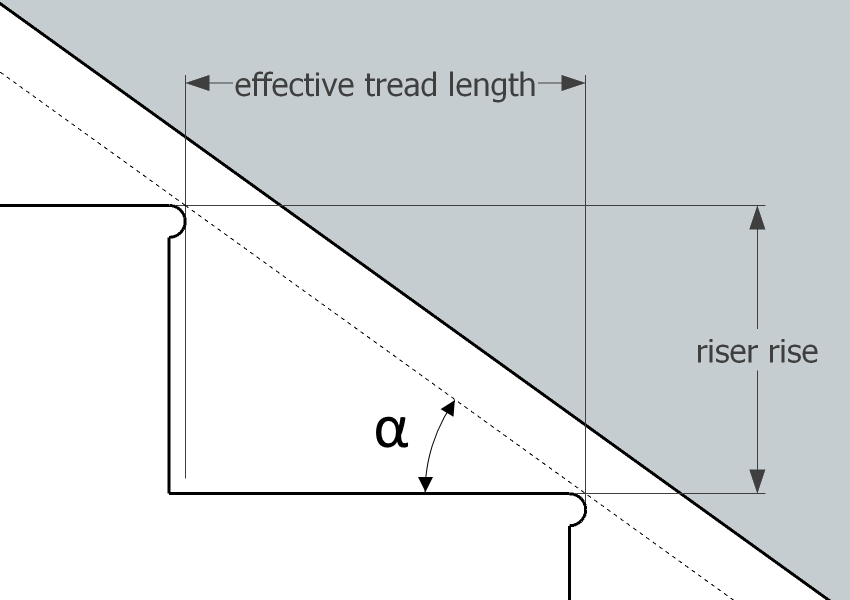# Spindle Spacing Calculator

Created by Kenneth Alambra
Reviewed by Steven Wooding
Last updated: Nov 19, 2022

This spindle spacing calculator will help you determine the recommended spacing between your railing spindles when constructing stair or deck railings. With this spindle calculator, you will get even uniform spindle spacings, not just between each spindle but also between its supporting walls or posts.

In this stair and deck spindle calculator, you will learn:

• What deck and stair spindles are, and why we need them;
• How to use our spindle spacing calculator;
• How to calculate spindle spacing yourself using two methods; and
• How to calculate slanted spindle spacing after finding its corresponding horizontal measurement.

🙋 Please note that when using this tool as a stair spindle calculator, we assume you will install the spindles on a base or shoe railing and not directly on the stair treads.

## What are deck and stair spindles?

Spindles, in construction and carpentry, are slender support members of a structure used to create a see-through barrier, like a fence, for staircases and bridges or an open parapet for elevated surfaces like decks, terraces, and balconies. We usually install spindles vertically from a bottom base (i.e., the floor or a base or shoe rail) to the underside of a handrail or a guard rail.These railings, at adequate heights, offer guidance and protection for people from accidentally falling over and off the aforementioned elevated surfaces. However, we also want to protect people, especially children, from passing through the spaces between the spindles.

Because of that, we want to space our spindles properly and only allow a maximum spindle spacing of 10 cm or 4 inches, as mandated by the U.S. Building Code. That is a safe measurement to prevent a small child's head from passing through or getting stuck between spindles.

This calculator will help you decide how to space your spindles to pass your local code and have beautiful and evenly spaced spindles when done correctly.

🔎 Balusters, which are similar objects to spindles, have the same functions as spindles. However, spindles are usually more slender versions of balusters. We also usually see spindles made from wood or metal materials. While most of the time, balusters are made from wood, stone, or cement. You can learn more about balusters in our baluster calculator.

If you need to install an entire fence and don't know where to start, perhaps our fence calculator can be your introduction. 🙂

## How to use this spindle spacing calculator

Our spindle spacing calculator is an easy and intuitive tool to use. Nevertheless, here are the steps you can follow while you use it:

1. Choose what you want to calculate, whether you wish to calculate spindle spacing for stairs or a deck. For deck installations, you can check our decking calculator for more information.
2. Select the spacing option you want to display. Pick centered spindles if you're going to space your spindles equally and find their balanced end spacings. Choose evenly-spaced spindles if you want to have an even spindle spacing throughout the railing. If you want to compare the results from both options, select both spacing options.
3. Enter the inside railing distance or the distance between two walls or posts where you want to install a railing.
4. Input the width of the spindle you wish to use. If you plan to use spindles with an intricate design with varying widths, enter the minimum width of your spindle. The point at which you measure the spindle width shall coincide with the imaginary guiding line where you will be measuring the spindle spacing during the actual construction.
5. Enter your desired maximum allowable spacing for your spindles. However, please keep in mind the mandated building code in your area to keep your railings safe for kids.
6. If you selected to calculate stair spindle spacing, you can enter your stair's riser rise and effective tread run or the stair pitch to calculate the slanted spacing of your spindles. You can skip this step if you're using our tool as a deck spindle calculator.

Upon doing these steps, our calculator will immediately display the results for your selected spindle spacing option.

In the next section of this text, you'll understand how to figure out the spindle spacing yourself by considering an example. Ready? Then let's go! 🙂

## How to calculate spindle spacing

The best way to understand how to calculate spindle spacing is by considering an example. Let's say we want to use 2-cm wide spindles for a stair railing with a total horizontal railing length of 85 cm from an existing post to the outer side of a 10-cm square end post.

Our first step is to determine the inside railing distance or the "actual space" we'll consider for the calculation. We do that by subtracting the 10-cm width of the end post from the 85-cm railing length to get 75 cm, as shown in the illustration below:In other cases, we just measure the distance between existing walls or posts to get the actual space.

Then, we determine what we call the "unit length." Unit length is the sum of one space between two spindles and the width of a spindle. Say we only allow a maximum of 10 cm for the spindle spacing. Our unit length will then be:

\scriptsize \begin{align*} \text{unit length} &=w_\text{s} + s_\text{max}\\ &= 2\ \text{cm} - 10\ \text{cm}\\ &= 12\ \text{cm} \end{align*}

where:

• $w_\text{s}$Spindle width; and
• $s_\text{max}$Maximum allowable or desired spindle spacing.

Next, we divide the inside railing distance, $d$, by the unit length to find how many unit lengths can fit in our actual space. By considering only the whole number of the quotient we will get, we also determine the number of spindles, $n$ for our railing. In equation form, we have:

\scriptsize \begin{align*} n &= \frac{d}{\text{unit length}}\\\\ &= \frac{75\ \text{cm}}{12\ \text{cm}}\\\\ &= 6\tfrac{1}{4} \text{unit lengths}\ \text{or}\ 6\ \text{spindles} \end{align*}

That means we can fit 6 of the 12-cm unit length plus an end spacing of 1/4 of a unit length, or 3 cm, within our actual space. We can also interpret that to say that we will need six spindles for this area, as we can see in this illustration:However, it looks better to center our six spindles so that the distance between the first spindle and the existing post is equal to the distance between the last spindle and the end post. For that, we have to take the average of these end spacings to get balanced end spacings. To do that:

1. We first multiply our unit length of 12 cm by 6 to get 72 cm.
2. Then we subtract that 72 cm from 75 cm to obtain the extra space of 3 cm.
3. Finally, we add 3 cm to 10 cm and divide their sum by 2 to get 6.5 cm for the balanced end spacing.

We can also express that in equation form as shown below:

\scriptsize \begin{align*} s_\text{end} &= \frac{d - n\ \times \text{unit length} + s_\text{max}}{2}\\\\ &= \frac{75\ \text{cm} - 6\ \times 12\ \text{cm} + 10\ \text{cm}}{2}\\\\ &= \frac{75\ \text{cm} - 72\ \text{cm} + 10\ \text{cm}}{2}\\\\ &= \frac{3\ \text{cm} + 10\ \text{cm}}{2}\\\\ &= \frac{13\ \text{cm}}{2}\\\\ &= 6.5\ \text{cm}\\ \end{align*}

where:

• $s_\text{end}$Balanced end spacing between the end spindles to the wall or post closest to them.

Now that we know our balanced end spacing, we now have the option to install our first spindle 6.5 cm from the previously installed post and then space the following five spindles 10 cm from each other. Following this procedure will leave us with our last spindle 6.5 cm away from our newly installed post, as we can see in the illustration below:Now that looks better! 🙂

On the other hand, if we want to have even spindle spacing throughout the entire railing, we need to calculate the spacing between the spindles differently. Let's learn how to do that in the next section of this text.

## Spindle spacing formula for evenly-spaced spindles

After knowing the number of unit lengths, $n$, that would fit our actual space, here is how to calculate the spacing for evenly-spaced spindles:

1. We first determine how much space all our spindles take by multiplying the width of our spindles by $n$ to get: 2 cm per spindle × 6 spindles = 12 cm.
2. Then, we subtract that from our actual space to obtain 75 cm - 12 cm = 63 cm. That is all the spaces between our spindles combined into one.
3. Since we have 6 unit lengths and one extra space at the end, we have to divide 63 cm by 7 spaces to get the value for an even spindle spacing of 9.0 cm.

We can also use the more general spindle spacing formula that does the same calculation but faster. Here we use the general spindle spacing formula to calculate the same result as explained earlier:

\scriptsize \begin{align*} s_\text{even} &= \frac{d - (n\ \times w_\text{s})}{n + 1}\\\\ &= \frac{75\ \text{cm} - (6\ \times 2\ \text{cm})}{6 + 1}\\\\ &= \frac{75\ \text{cm} - (12\ \text{cm})}{7}\\\\ &= \frac{63\ \text{cm}}{7}\\\\ &= 9.0\ \text{cm} \end{align*}

where:

• $s_\text{even}$Even spindle spacing throughout the railing.

With this option, we can install the first spindle 9.0 cm from the existing post, install the next spindle 9.0 cm away from the first spindle, and so on. After installing the sixth spindle, the spacing between the sixth spindle and the newly installed post should also be around 9.0 cm, as shown below:## Slanted spindle spacing

Learning how to figure out the spindle spacing along a slanted surface like a base rail also comes in handy in some situations (e.g., when using a spindle design with varying diameters or widths for a stair railing, when determining the length of fillet strips). Here is an illustration showing an example of a slanted spindle spacing:After calculating the horizontal spacing of your spindles by following the instructions described above, we can determine the spacing between spindles along a slanted base rail or a diagonal guideline using this formula:

$\footnotesize{S_\text{slanted} = \frac{S_\text{horizontal}}{\cos(\alpha)}}$

where:

• $S_\text{slanted}$Slanted spacing along the base or shoe rail;
• $S_\text{horizontal}$Calculated spacing along the horizontal; and
• $\alpha$Base rail angle of inclination equivalent to the stair pitch.

If you have a protractor, you can use that to determine the base rail angle of inclination. We can also calculate it if you don't have any angle-measuring tool right now. Since it would be prettier to make the base rail parallel to the stair's angle of inclination, we can use this stair pitch formula if we need to calculate $\alpha$:

$\footnotesize{\alpha = \tan^{-1}\left(\frac{\text{riser rise}}{\text{effective tread run}}\right)}$

where:

• $\small{\text{riser rise}}$Vertical distance between two adjacent steps; and
• $\small{\text{effective tread run}}$Horizontal distance from two same points on two adjacent steps (e.g., the horizontal distance from the two adjacent stair nosing tips).Though our spindle calculator can do these steps very quickly, wasn't it fun to learn how to calculate the spacing between spindles ourselves? 🙂 Now that we have all the measurements we need, we can now proceed to the actual construction of our railing 👷‍♂️👷‍♀️.

💡 Aside from calculating spindle spacing for stairs and deck railings, you can also use this tool and these methods for determining spindle spacings for your other woodworking projects like, let's say, a chair's backseat or a baby crib 👶.

## FAQ

### What is the maximum spacing for spindles?

The maximum spacing for spindles is around 10 cm or 4 inches. We consider this maximum allowed spindle spacing to keep our railings safe by preventing a child's head from getting stuck between spindles. A home inspector may bring a 4-in ball to check if your spindles are up to code.

### How many spindles do I need?

The number of spindles you need depends on your:

• Spindle width;
• Inside railing distance; and
• Maximum allowed spindle spacing.

Let's say you need to install 3-cm wide spindles between two posts 200 cm apart, and you want to space them 10 cm max.

1. Add the spindle width and max allowed spacing: 3 cm + 10 cm = 13 cm.
2. Divide the inside railing distance of 200 cm by 13 cm to get: 200 cm / 13 cm = 15.38.
3. Round down this result to a whole number. In this case 15 spindles.

### How do I evenly space spindles?

To evenly space spindles quickly:

1. Multiply your spindle's width by the number of spindles you need to find the total width of all your spindles. Say, 15 spindles × 3 cm = 45 cm.
2. Subtract your spindles' total width from the railing length you will install them to get the total width of all the combined spaces. Say, 200 cm - 45 cm = 155 cm.
3. Divide this difference by the number of spaces you need, usually the number of spindles plus one. Therefore, we have: 155 cm / (15 + 1) ≈ 9.68 cm.

### Can spindles be horizontal?

Spindles can be horizontal. However, horizontal spindles can pose a danger since people can use them as a ladder to climb the railings. Please check your local building code to see if they allow homeowners in your area to install horizontal spindles.

Kenneth Alambra
Calculate spindle spacing for...
a deck.
Spacing option
Evenly-spaced spindles
Railing details
Inside railing distance
ft
Spindle width
in
Max. allowable spacing
in
Evenly-spaced spindles
Number of spindles
spindles
Spindle spacing
in
People also viewed…

### Drywall

The drywall calculator determines the number of drywall panels you need to install in a room.

### Epoxy

Our epoxy calculator will help you find the right amount of epoxy resin required to coat your desired surface.

### Korean age

If you're wondering what would your age be from a Korean perspective, use this Korean age calculator to find out.

### Sleep

The sleep calculator can help you determine when you should go to bed to wake up happy and refreshed.